﻿ A General Form of Fuzzy Linear Fractional Programs with Trapezoidal Fuzzy Numbers

### A General Form of Fuzzy Linear Fractional Programs with Trapezoidal Fuzzy Numbers

Sapan Kumar Das, S. A. Edalatpanah

## A General Form of Fuzzy Linear Fractional Programs with Trapezoidal Fuzzy Numbers

Sapan Kumar Das1,, S. A. Edalatpanah2

1Department of Mathematics, National Institute of Technology Jamshedpur, India

2Department of Applied Mathematics, Lahijan Branch, Islamic Azad University, Lahijan, Iran

### Abstract

In this paper, we have been pointed out the study of fuzzy linear fractional programming (FLFP) problems with trapezoidal fuzzy numbers. Where the objective functions are fuzzy numbers and the constraints are real numbers. In this paper a new efficient method for FLFP problem has been proposed, in order to obtain the fuzzy optimal solution with unrestricted variables and parameters. These proposed methods is based on crisp linear fractional programming and newly transformation technique also used. A computational procedure has been presented to obtain an optimal solutions. To show the efficiency of our proposed method a real life example has been illustrated.

• Sapan Kumar Das, S. A. Edalatpanah. A General Form of Fuzzy Linear Fractional Programs with Trapezoidal Fuzzy Numbers. International Journal of Data Envelopment Analysis and *Operations Research*. Vol. 2, No. 1, 2016, pp 16-19. http://pubs.sciepub.com/ijdeaor/2/1/3
• Das, Sapan Kumar, and S. A. Edalatpanah. "A General Form of Fuzzy Linear Fractional Programs with Trapezoidal Fuzzy Numbers." International Journal of Data Envelopment Analysis and *Operations Research* 2.1 (2016): 16-19.
• Das, S. K. , & Edalatpanah, S. A. (2016). A General Form of Fuzzy Linear Fractional Programs with Trapezoidal Fuzzy Numbers. International Journal of Data Envelopment Analysis and *Operations Research*, 2(1), 16-19.
• Das, Sapan Kumar, and S. A. Edalatpanah. "A General Form of Fuzzy Linear Fractional Programs with Trapezoidal Fuzzy Numbers." International Journal of Data Envelopment Analysis and *Operations Research* 2, no. 1 (2016): 16-19.

 Import into BibTeX Import into EndNote Import into RefMan Import into RefWorks

### 1. Introduction

Linear fractional programming (LFP) problem is one of the most important techniques in operation research. Many real world problem can be transformed to linear fractional programming model. Hence this model is an indispensable tool for today’s applications such asfinancial sector, hospitality, industrial sector etc. It is a mathematical technique for optimal allocation to several activities on the basis of given decision of optimality. This type of problem is evidently an uncertain optimization problem due to its decision based system. So, it lead to propose a new concept in fuzzy optimization by Bellman and Zadeh . Lotfi et al.  introduced a method to obtain the approximate the solution of fully fuzzy linear programming problems. Amit Kumar et al.  proposed a method for solving fully fuzzy linear programming problems using idea of crisp linear programming and ranking function.

Recently, Veeramani and Sumathi  established a new method for solving fuzzy linear fractional Programming problem and they have transformed the problem into a multi objective linear programming problem. Ganesan and Veeramani  introduced the fuzzy linear programming problem with trapezoidal fuzzy numbers without converting them to crisp linear programming problem.A. Ebrahimnejad and M. Tavana  proposed a new concept which the coefficients of objective function and the values of the right hand side are represented by trapezoidal fuzzy numbers and other parts are represented by real numbers. They convert the fuzzy linear programming problem into an equivalent crisp linear programming problem and solved by simplex method. Sapan Das et al.  proposed a method to solving fuzzy linear fractional programming problem with multi-objective linear programming problem. Again Sapan Das et al.  proposed a method for solving fully fuzzy linear fractional programming problem with triangular fuzzy numbers.

In this paper, a new method is proposed for finding the fuzzy optimal solution of FLFP problems with inequality constraints. The coefficients of the objective function are represented by trapezoidal fuzzy numbers and the constraints are represented by real numbers. We introduce a new type of fuzzy arithmetic for symmetric trapezoidal fuzzy numbers and propose a method for solving fuzzy linear fractional programming problem with converting them to crisp linear fractional programming problems. After that, we used a new transformation technique used to solve these crisp linear fractional programming problem. To illustrate the proposed method, numerical examples are solved.

This paper is organized as follows: In section 2 some basic definitions of fuzzy symmetric trapezoidal fuzzy number and some arithmetic results also. In section 3, formulation of FLFP problems and application of ranking function for solving FLFP problems are established. A new method is proposed for solving FLFP problems in section 4. In section 5, we give a numerical example involving symmetrical trapezoidal fuzzy numbers to illustrate the theory developed in this paper. Finally, in Section 6 we present the conclusion part.

### 2. Preliminaries

The aim of this section is to present some notations and results of fuzzy set theory are discussed.

Definition 2.1 . A convex fuzzy set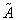on R is a fuzzy number if the following conditions hold:

(a) Its membership function is piecewise continuous.

(b) There exist three intervals [a, b], [b, c] and [c, d] such that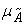is increasing on [a, b], equal to 1 on [b, c], decreasing on [c, d] and equal to 0 elsewhere.

Definition 2.2 . The arithmetic operations on two symmetric trapezoidal fuzzy numbers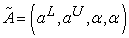and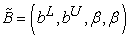are given by: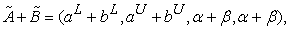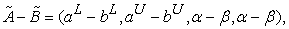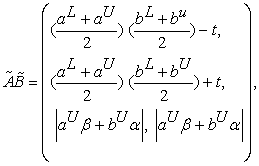Where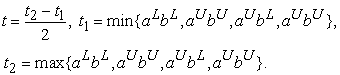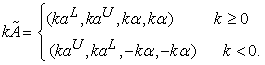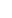Definition 2.3 . Let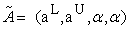and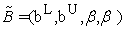be two symmetric trapezoidal fuzzy numbers. The relations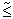and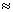are defined as follows:

(i)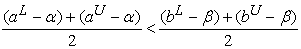, that is(in this case, we may write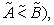or

(ii)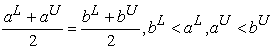(in this case we say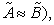or

(iii)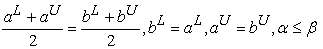(in this case we say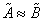).

Definition 2.4  A fuzzy seton R is called a symmetric trapezoidal fuzzy number if its membership function is defined as follows: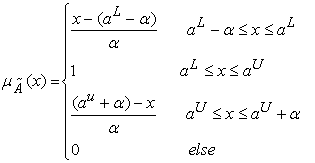### 3. Proposed Method to Convert LFP into LP

In this section we reefer  a transformation method to convert linear fractional to equivalent linear programming as follows:

Transformation of Objective: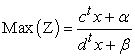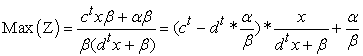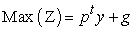Where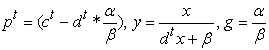Transformation of constraints: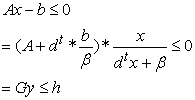Where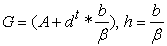Now the above transformation we get the linear programming problem as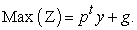Subject to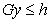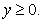### 4. Proposed Method to Find the Fuzzy Optimal Solution of FLFP Problems

In this section, a new method is proposed to find the fuzzy optimal solution of the following type of fuzzy linear fractional programming (FLFP) problems: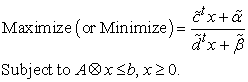(1)

Where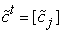is 1 by n matrix;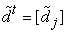is 1 by n matrix;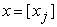is n by 1 matrix;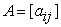is m by n matrix;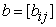is a m by 1 matrix;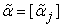and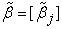are the scalars.

Based on above definition 2.3, we define a rank for each symmetric trapezoidal fuzzy number for comparison purposes. Assuming that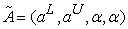is a symmetric trapezoidal fuzzy number, then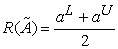. This equation allows us to convert the fuzzy linear fractional programming (FLFP) problem in to a crisp linear fractional programming (CLFP) problem. We substitute the rank order of each fuzzy number for the corresponding fuzzy number in the fuzzy problem under consideration. This leads to an equivalent crisp linear fractional programming problem which can be solved by standard method. After we have got the crisp linear fractional programming we used a new transformation method for converting into an equivalent crisp linear programming problem and solved by simplex method.

In the following we are going to introduce algorithm to find an exact optimal solution of FLFP problem. The steps of the proposed algorithm are given as follows:

Step 1. With respect to the general form of our problem can be written as follows: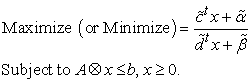(2)

Step 2. Regarding definitions 2.3, the problem (2) is converted to the crisp linear fractional programming problem may be written as follows:(3)

Step 3. In terms of objective function the transformation method will be used into an equivalent crisp linear programming problem so we have,(4)

Step 4. Solve the problem (4) with the help of Lingo software: we get the optimal solution.

### 5. Numerical Example

In this section, we illustrate the proposed algorithm using a real life problem. Linear fractional programming problem is evidently an uncertain optimization problem due to its variations in the maximum daily requirements. So, the amount of each product of ingredient will be uncertain. Hence, we will model the problem as a Fuzzy linear fractional programming (FLFP) problem. We use trapezoidal fuzzy numbers for each uncertain value. Also, the mathematical programming problem will be solved by Lingo.

Example. (Production Planning)

In Jamshedpur City, India, A Wooden company is the producer of two kinds of products A and B with profit around (4, 6, 3,3) and around (1, 5, 1, 1) dollar per unit, respectively. However the cost for each one unit of the above products is around (3, 7, 2, 2) and around (3, 1, 1, 1) dollars respectively. It is assume that a fixed cost of around (1, 1, 2, 2) dollar is added to the cost function due to expected duration through the process of production. Suppose the raw material needed for manufacturing product A and B is about 3 unitsper pound and about 5 units per pound respectively, the supply for this raw material is restricted to about 15 pounds. Man-hours per unit for the product A is about 5 hour and product B is about 2 hour per unit for manufacturing but total Man-hour available is about 10 hour daily. Determine how many products A and B should be manufactured in order to maximize the total profit.

This real life problem can be formulated to the following FLFP problem: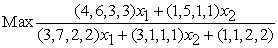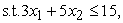(5)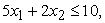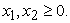So, with respect to Step 2 we convert the problem (2) into an equivalent crisp linear programming problem as follows: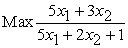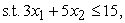(6)Now, the crisp linear fractional programming problem (6) converting into an equivalent crisp linear programming problem by using Step 3.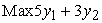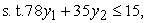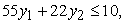(7)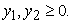The problem (7) is the crisp linear programming problem. Now solved by simplex method we get the result is: y1=0, y2=0.42, and the objective function value is Z=1.28.

by comparing the result of proposed method with Veeramani and Sumathi method et al. , we conclude that our result is more effective, because: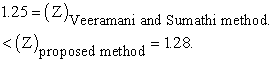Example 2: A company manufactures two kinds of products A and B with profit around 5 and around 3 dollar per unit, respectively. However the cost for each one unit of the above products is around 5 and around 2 dollars respectively. It is assume that a fixed cost of around 1 dollar is added to the cost function due to expected duration through the process of production. Suppose the raw material needed for manufacturing product A and B is about 3 unitsper pound and about 5 units per pound respectively, the supply for this raw material is restricted to about 15 pounds. Man-hours per unit for the product A is about 5 hour and product B is about 2 hour per unit for manufacturing but total Man-hour available is about 10 hour daily. Determine how many products A and B should be manufactured in order to maximize the total profit.

Solution: Let y1 and y2to be the amount of units of A and B to be produced. Then the above problem can be formulated as: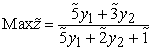Subject to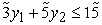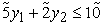Now we take the coefficients of variables as follows: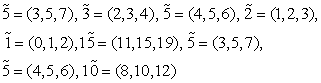Now the problem can be written as: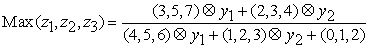Subject to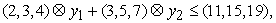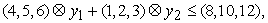we solve this problem by using our proposed method and compare with exciting methods:

Step 13 We get the fuzzy optimal solution of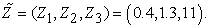In Veeramani and Sumathi method, the fuzzy optimal solution is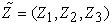=(0.53,1.27,9).

By comparing the results of proposed method with Veeramani and Sumathi method, based on definition 2.7, we can conclude that our result is more effective, because: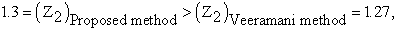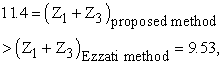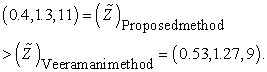### 6. Conclusion

In the past few years, a growing interest has been shown in Fuzzy linear fractional programming and currently there are several methods for solving FLFP problem. In this paper, a new efficient method has been proposed, in order to obtain the fuzzy optimal solution of fuzzy linear fractional programming problems with inequality constraints occurring in daily real life problem. We showed that the method proposed in this paper is very reliability and applicability. To illustrate the proposed method two real life numerical examples are solved.

### References

  Ebrahimnejad, M. Tavana, A novel method for solving linear programming problems with symmetric trapezoidal fuzzy numbers, Appl. Math. Model. 38 (7). (2014). 4388-4395.In article View Article  Kumar, J. Kaur, P. Singh, A new method for solving fully fuzzy linear programming problems, Appl. Math. Model. 35. (2011). 817-823.In article View Article  F. H. Lotfi, T.Allahviranloo, M.A. Jondabeha, L. Alizadeh, Solving a fully fuzzy linear programming using lexicography method and fuzzy approximate solution, Appl. Math. Model. 33. (2009). 3151-3156.In article View Article  H. R. Maleki, M. Tata, M. Mashinchi, Fuzzy number linear programming, ProcInternat Conf. on intelligent and Cognitive Systems FSS Sponsored by IEE and ISRF, Tehran, Iran. (1996). 145-148.In article  Veeramani, M. Sumathi, Fuzzy mathematical programming approach for solving fuzzy linear fractional programming problem, RAIRO Operation Research 48. (2014). 109-122.In article View Article  H. Tanka, T. Okuda, K. Asai, On fuzzy mathematical programming, J. Cybern, 3(4). (1974). 37-46.In article View Article  H.J. Zimmerman, Fuzzy programming and linear programming with several objective functions, Fuzzy Sets Syst. 1(1). (1978). 45-55.In article View Article  H.J. Zimmermann, Trends and new approaches in European operational research, J. Opl. Res. Soc. 33. (1982). 597-603.In article View Article  S. K. Das, T. Mandal, A single stage constraints linear fractional programming problem: An Approach, Operation Research and Applications: An International Journal, 2. (2015). 1-5.In article  R. E. Bellman, L. A. Zadeh, Decision making in a fuzzy environment, Manage. Sci. 17(4). (1970). 141-164.In article View Article  Ganesan, P. Veer Amani, Fuzzy linear programs with trapezoidal fuzzy numbers, Ann. Oper. Res. 143. (206). 305-315.In article  Sapan K.Das, T. Mandal, and S. Edalatpanah, A note on “A new method for solving fully fuzzy linear fractional programming with a triangular fuzzy numbers”. App. Math. and Comp. Intel., 2015. 4(1): p. 361-367.In article  Sapan K. Das, T. Mandal, and S.A. Edalatpanah, A new approach for solving fully fuzzy linear fractional programming problems using the Multi objective linear programming problem RAIRO Oper. Res., 2016.In article  Sapan K. Das, T. Mandal , and S.A. Edalatpanah, A mathematical model for solving fully fuzzy linear programming problem with trapezoidal fuzzy numbers. Applied Intelligence, 2016: p. 1-11.In article